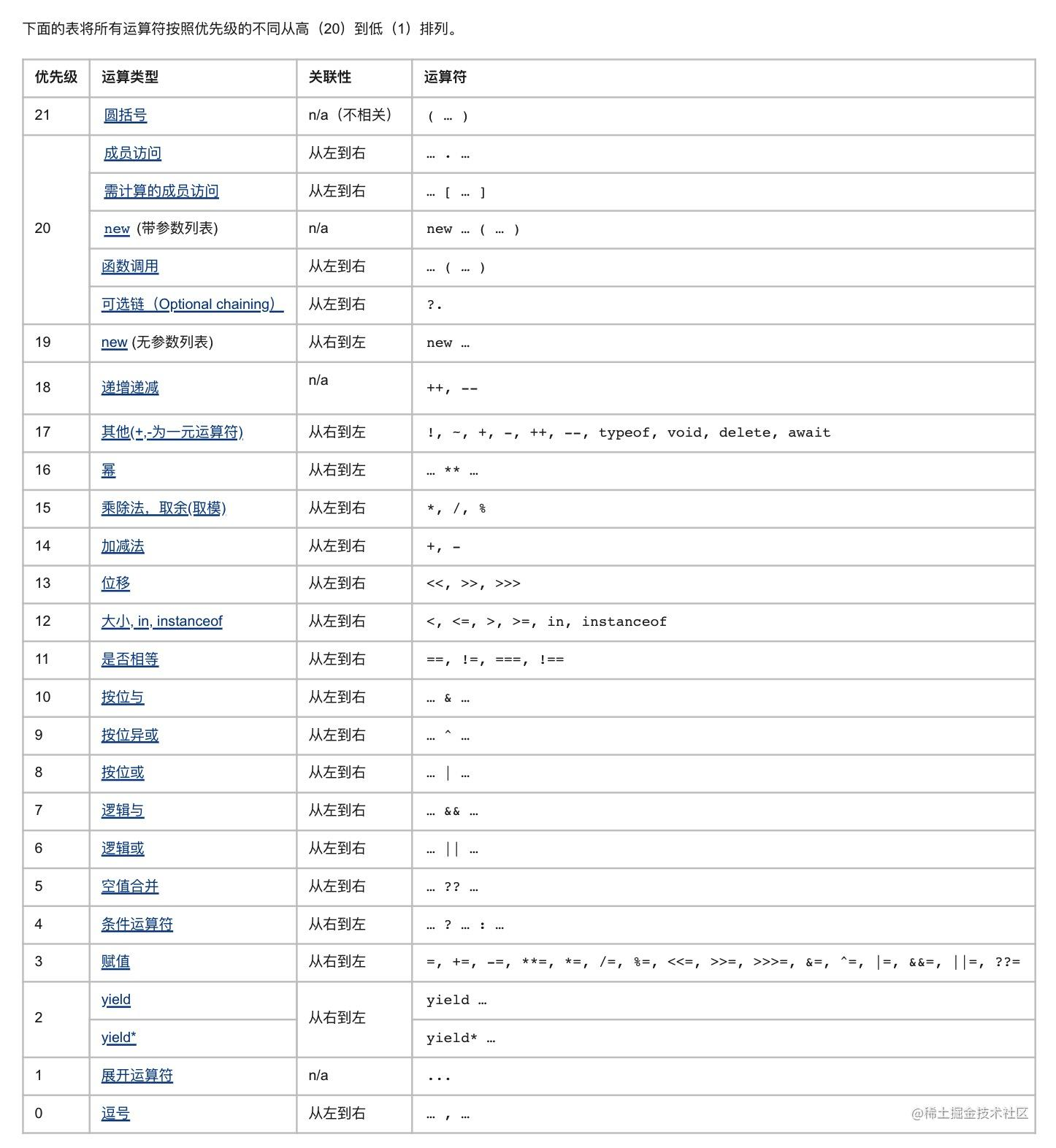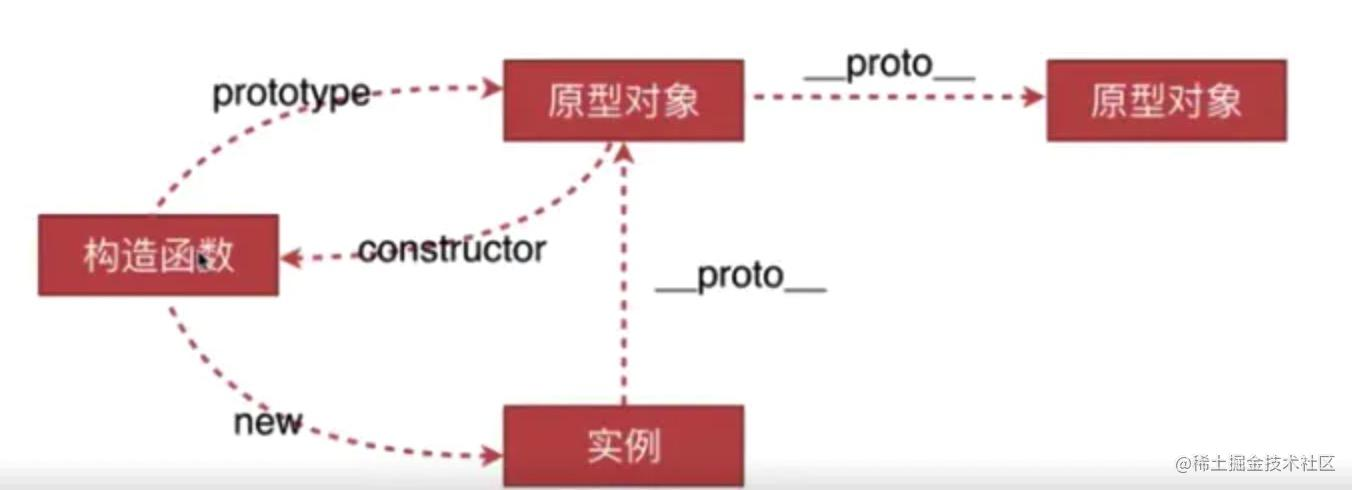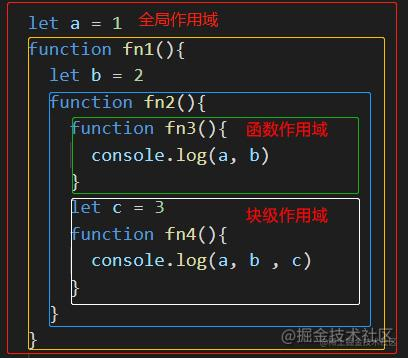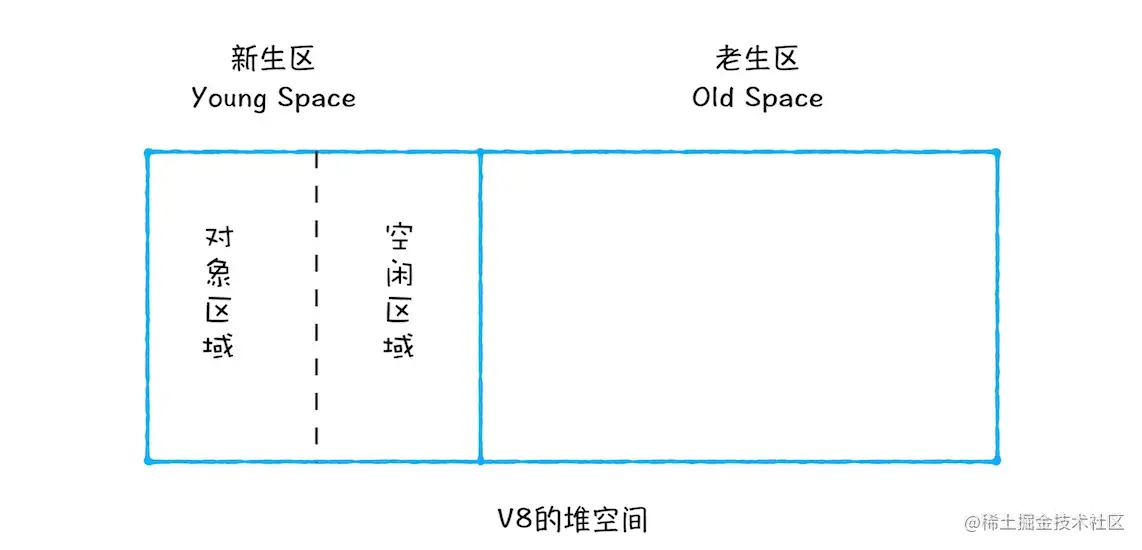# JavaScript进阶知识点## 自定义事件

``````// 注册事件  不可以添加参数
let eve1 = new Event("myClick")
// 可以添加参数
let eve2 = new CustomEvent('myClick',params)

// 监听事件
console.log("myClick")
})

// 触发事件
dom.dispatchEvent(eve1)

## Set、Map

`Set``Map` 都是强引用(下面有说明)，都可以遍历，比如 for of / forEach

Set

``````const set1 = new Set()
const set2 = new Set([1,2,3])
console.log(set1) // { 1 }

console.log(set2) // { 1, 2, 3, '沐华' }
console.log(set2.size) // 4
console.log(set2.has('沐华')) // true
set2.delete('沐华')
console.log(set2) // { 1, 2, 3 }

// 用 Set 去重

const set3 = new Set([1, 2, 1, 1, 3, 2])
const arr = [...set3]
console.log(set3) // { 1, 2, 3 }
console.log(arr) // [1, 2, 3]

// 引用类型指针不一样，无法去重
const set4 = new Set([1, { name: '沐华' }, 1, 2, { name: '沐华' }])
console.log(set4) // { 1, { name: '沐华' }, 2, { name: '沐华' } }

// 引用类型指针一样，就可以去重
const obj = { name: '沐华' }
const set5 = new Set([1, obj, 1, 2, obj])
console.log(set5) // { 1, { name: '沐华' }, 2 }

Map

``````const map1 = new Map()
map1.set(0, 1)
map1.set(true, 2)
map1.set(function(){}, 3)
const map2 = new Map([ [0, 1], [true, 2], [{ name: '沐华' }, 3] ])
console.log(map1) // {0 => 1, true => 2, function(){} => 3}
console.log(map2) // {0 => 1, true => 2, {…} => 3}

console.log(map1.size) // 3
console.log(map1.get(true)) // 2
console.log(map1.has(true)) // true
map1.delete(true)
console.log(map1) // {0 => 1, function(){} => 3}

## WeakSet、WeakMap

`WeakSet``WeakMap` 都是弱引用，对 `GC` 更加友好，都不能遍历

WeakSet

``````const ws1 = new WeakSet()
let obj = { name: '沐华' }
console.log(ws1) // { function(){}, { name: '沐华' } }
console.log(ws1.has(obj)) // true

ws1.delete(obj)
console.log(ws1.has(obj)) // false

WeakMap

``````const wm1 = new WeakMap()

const o1 = { name: '沐华' },
o2 = function(){},
o3 = window

wm1.set(o1, 1) // { { name: '沐华' } : 1 } 这样的键值对
wm1.set(o2, undefined)
wm1.set(o1, o3); // value可以是任意值,包括一个对象或一个函数
wm1.set(wm1, wm2); // 键和值可以是任意对象,甚至另外一个WeakMap对象

wm1.get(o1); // 1 获取键值

wm1.has(o1); // true  有这个键名
wm1.has(o2); // true 即使值是undefined

wm1.delete(o1);
wm1.has(o1);   // false

## 类型检测

typeof

``````typeof 1 === "number" // true
typeof "a" === "string" // true
typeof true === "boolean" // true
typeof undefined === "undefined" // true
typeof Symbol() === "symbol" // true

``````typeof null === "object" // true
typeof {} === "object" // true
typeof [] === "object" // true
typeof function () {} === "function" // true

instanceof

``````console.log(null instanceof Object) // false
console.log({} instanceof Object) // true
console.log([] instanceof Object) // true
console.log(function(){} instanceof Object) // true

toString

``````let toString = Object.prototype.toString

console.log(toString.call(1)) // [object Number]
console.log(toString.call('1')) // [object String]
console.log(toString.call(true)) // [object Boolean]
console.log(toString.call(undefined)) // [object Undefined]
console.log(toString.call(null)) // [object Null]
console.log(toString.call({})) // [object Object]
console.log(toString.call([])) // [object Array]
console.log(toString.call(function(){})) // [object Function]
console.log(toString.call(new Date)) // [object Date]

``````// constructor  判断是否是该类型直接继承者
let A = function(){}
let B = new A()
A.constructor === Object // false
A.constructor === Function // true
B.constructor === Function // false
B.constructor === A // true

// 判断数组
console.log(Array.isArray([])) // true
// 判断数字
function isNumber(num) {
let reg = /^[0-9]+.?[0-9]*\$/
if (reg.test(num)) {
return true
}
return false
}

//封装获取数据类型
function getType(obj){
let type = typeof obj
if(type !== 'object'){
return type
}
return Object.prototype.toString.call(obj)
}

// 含隐式类型转换 继续往下看

// 判断数字
function isNumber(num) {
return num === +num
}
// 判断字符串
function isString(str) {
return str === str+""
}
// 判断布尔值
function isBoolean(bool) {
return bool === !!bool
}

## 类型转换

JS没有严格的数据类型，所以可以互相转换

• 显示类型转换：`Number()`, `String()`, `Boolean()`
• 隐式类型转换：四则运算，判断语句，`Native` 调用，`JSON` 方法

1. Number()

``````console.log(Number(1)) // 1
console.log(Number("1")) // 1
console.log(Number("1a")) // NaN
console.log(Number(true)) // 1
console.log(Number(undefined)) // NaN
console.log(Number(null)) // 0
console.log(Number({a:1})) // NaN 原因往下看

• 数字：转换后还是原来的值
• 字符串：如果能被解析成数字，就得到数字，否则就是 `NaN`，空字符串为0
• 布尔值：`true` 转为1，false 转为0
• `undefined`: 转为 `NaN`
• `null`：转为0 引用类型转换
``````let a = {a:1}
console.log(Number(a)) // NaN
// 原理
a.valueOf() // {a:1}
a.toString() // "[object Object]"
Number("[object Object]") // NaN

• 先调用对象自身的 `valueOf` 方法，如果该方法返回原始类型的值(数值、字符串和布尔值)，则直接对该值使用 `Number` 方法，不再继续
• 如果 `valueOf` 方法返回复合类型的值，再调用对象自身的 `toString` 方法，如果 `toString` 方法返回原始类型的值，则对该值使用 `Number` 方法，不再继续
• 如果 `toString` 方法返回的还是复合类型的值，则报错

2. String()

``````console.log(String(1)) // "1"
console.log(String("1")) // "1"
console.log(String(true)) // "true"
console.log(String(undefined)) // "undefined"
console.log(String(null)) // "null"
console.log(String({b:1})) // "[object Object]" 原因往下看

• 数字：转换成相应字符串
• 字符串：转换后还是原来的值
• 布尔值：`true` 转为"true"，`false` 转为"false"
• `undefined`: 转为"undefined"
• `null`：转为"null" 引用类型转换
``````let b = {b:1}
console.log(String(b)) // "[object Object]"
// 原理
b.toString() // "[object Object]"
// b.valueOf() 由于返回的不是复合类型所以没有调valueOf()
String("[object Object]") // "[object Object]"

• 先调用 `toString` 方法，如果 `toString` 方法返回的是原始类型的值，则对该值使用 `String` 方法，不再继续
• 如果 `toString` 方法返回的是复合类型的值，再调用 `valueOf` 方法，如果 `valueOf` 方法返回的是原始类型的值，则对该值使用 `String` 方法，不再继续
• 如果 `valueOf` 方法返回的是复合类型的值，则报错

3. Boolean()

``````console.log(Boolean(0)) // flase
console.log(Boolean(-0)) // flase
console.log(Boolean("")) // flase
console.log(Boolean(null)) // flase
console.log(Boolean(undefined)) // flase
console.log(Boolean(NaN)) // flase

• 0
• -0
• ""
• null
• undefined
• NaN

``````// 四则运算  如把String隐式转换成Number
console.log(+'1' === 1) // true

// 判断语句  如把String隐式转为Boolean
if ('1') console.log(true) // true

// Native调用  如把Object隐式转为String
console.log(([][[]]+[])[+!![]]+([]+{})[!+[]+!![]]) // "nb"

// JSON方法 如把String隐式转为Object
console.log(JSON.parse("{a:1}")) // {a:1}

``````console.log( true+true  ) // 2                   解：true相加是用四则运算隐式转换Number 就是1+1
console.log(  1+{a:1}   ) // "1[object Object]"  解：上面说了Native调用{a:1}为"[object Object]"  数字1+字符串直接拼接
console.log(   []+[]    ) // ""                  解：String([]) =》 [].toString() = "" =》 ""+"" =》 ""
console.log(   []+{}    ) // "[object Object]"   解："" + String({}) =》 "" + {}.toString() = "" + "[object Object]" =》 "[object Object]"
console.log(   {}+{}    ) // "[object Object][object Object]" 和上面同理## this

• 全局作用域、自执行函数、定时器传进的非箭头函数的 this 都指向 `window`
• 严格模式(`use strict`)下全局作用域中的 this 指向 `undefined`
• 构造函数中的this指向当前的实例
• 事件绑定函数中的this指向当前被绑定的元素
• 箭头函数中this指向定义箭头函数的上级作用域中的this

• 使用 `call`, `apply`, `bind`，call 和 apply 改变 this 指向时，函数会立即执行，bind 不会
• 保存成变量(`let self = this`)
• 使用箭头函数
• 使用 `new` 实例化一个对象
• 严格模式下直接调用函数 this 指向 undefined

## 箭头函数

• 箭头函数写法更简洁
• 箭头函数本身没有 this，所以没有 prototype
• 箭头函数不支持 new
• 箭头函数的 this 继承自外层第一个作用域的 this, 严格和非严格模式下都一样，修改被继承的this指向，那么箭头函数的 this 指向也会跟着改变
• 箭头函数指向全局时，arguments 会报错，否则 arguments 继承自外层作用域
• 箭头函数不支持函数形参重名

## 闭包

• 内部函数有权访问外部函数的局部变量

• 内部函数引用的变量会在内存中，不会立刻销毁;
• 因为内部函数有权访问外部函数，所以外部函数执行完了也不会被垃圾回收，而占用内存;
• 如果闭包用得太多会导致性能降低

## 浅拷贝

``````// 1. 展开运算符 ...
let obj1 = { a:1, b:{ c:3 } }
let obj2 = { ...obj1 }
obj1.a = 'a'
obj1.b.c = 'c'
console.log(obj1) // { a:'a', b:{ c:'c' } }
console.log(obj2) // { a:1, b:{ c:'c' } }

// 2. Object.assign() 把obj2合并到obj1
Object.assign(obj1, obj2)

// 3. 手写
function clone(target){
let obj = {}
for(let key in target){
obj[key] = target[key]
}
return obj
}

// 4. 数组浅拷贝  用Array方法 concat()和slice()
let arr1 = [ 1,2,{ c:3 } ]
let arr2 = arr1.concat()
let arr3 = arr1.slice()

## 深拷贝

1. JSON.parse(JSON.stringify())

``````let obj1 = { a:1, b:{ c:3 } }
let obj2 = JSON.parse(JSON.stringify(obj1))
obj1.a = 'a'
obj1.b.c = 'c'
console.log(obj1) // { a:'a', b:{ c:'c' } }
console.log(obj2) // { a:1, b:{ c:3 } }

2. 手写递归

``````function clone(target, map = new Map()){
if (typeof target === 'object') { // 引用类型才继续深拷贝
let obj = Array.isArray(target) ? [] : {} // 考虑数组
//防止循环引用
if (map.get(target)) {
return map.get(target) // 有拷贝记录就直接返回
}
map.set(target,obj) // 没有就存储拷贝记录
for (let key in target) {
obj[key] = clone(target[key]) // 递归
}
return obj
} else {
return target
}
}

• `WeakMap`替代`Map`，上面说了`WeakMap`是弱引用，`Map`是强引用
• 选择性能更好的循环方式

for in 每次迭代操作会同时搜索实例和原型属性，会产生更多的开销，所以用 while

``````// 用while来实现一个通用的forEach遍历
function forEach(array, iteratee) {
let index = -1;
const length = array.length;
while (++index < length) {
iteratee(array[index], index);
}
return array;
}
// WeakMap 对象是键/值对集合，键必须是对象，而且是弱引用的，值可以是任意的
function clone(target, map = new WeakMap()){
// 引用类型才继续深拷贝
if (target instanceof Object) {
const isArray = Array.isArray(target)
// 克隆对象和数组类型
let cloneTarget = isArray ? [] : {}

// 防止循环引用
if (map.get(target)) {
// 有拷贝记录就直接返回
return map.get(target)
}
// 没有就存储拷贝记录
map.set(target,cloneTarget)

// 是对象就拿出同级的键集合  返回是数组格式
const keys = isArray ? undefined : Object.keys(target)
// value是对象的key或者数组的值 key是下标
forEach(keys || target, (value, key) => {
if (keys) {
// 是对象就把下标换成value
key = value
}
// 递归
cloneTarget[key] = clone(target[key], map)
})
return cloneTarget
} else {
return target
}
}

## new

new 干了什么？

• 创建一个独立内存空间的空对象
• 把这个对象的构造原型(`__proto__`)指向函数的原型对象`prototype`，并绑定this
• 执行构造函数里的代码
• 如果构造函数有return就返回return的值，如果没有就自动返回空对象也就是this

``````function Person(name){
this.name = name
console.log(this) // { name: '沐华' }
return { age: 18 }
}
const p = new Person('沐华')
console.log(p) // { age: 18 }

## 原型``````function Parent(){} // 这就是构造函数
let child = new Parent() // child就是实例

Parent.prototype.getName = function(){ console.log('沐华') } // getName是构造函数的原型对象上的方法
child.getName() // '沐华' 这是继承来的方法

• `prototype` ：它是构造函数的原型对象。每个函数都会有这个属性，强调一下，是函数，其他对象是没有这个属性的
• `__proto__` ：它指向构造函数的原型对象。每个对象都有这个属性，强调一下，是对象，同样，因为函数也是对象，所以函数也有这个属性。不过访问对象原型(`child.__proto__`)的话，建议用`Es6``Reflect.getPrototypeOf(child)`或者`Object.getPrototypeOf(child)`方法
• `constructor` ：这是原型对象上的指向构造函数的属性，也就是说代码中的 `Parent.prototype.constructor === Parent` 是为 `true`

## 原型链

• 先访问对象的实例属性，找到就返回，没有就通过`__proto__`去原型对象中找
• 在原型对象上找到，就返回，没有继续通过原型的`__proto__`向上层查找
• 一直到`Object.prototype`，找到就返回，没有就返回`undefined`，不找了

## 原型污染

``````Object.prototype.toString = function () {alert('原生方法被改写，已完成原型污染')};

1. `Object.freeze(obj)`冻结对象，然后就不能被修改属性，变成不可扩展的对象
``````Object.freeze(Object.prototype)
Object.prototype.toString = 'hello'
console.log(Object.prototype.toString) // ƒ toString() { [native code] }

1. 不采用字面量形式，用`Object.create(null)`创建一个没有原型的新对象，这样不管对原型做什么扩展都不会生效
``````const obj = Object.create(null)
console.log(obj.__proto__) // => undefined

1. `Map` 数据类型，代替`Object`类型

`Map` 对象保存键/值对的集合。任何值（对象或者原始值）都可以作为一个键或一个值。所以用 Map 数据结构，不会对 Object 原型污染

Map 和 Object 不同点

• Object 的键只支持 String/Number/Symbol 两种类型，Map 的键可以是任意值，包括函数、对象、基本类型
• Map 中的键值是有序的，Object 中的键不是
• Map 在频繁增删键值对的场景下有性能优势
• 用 size 属性直接获取一个Map的键值对个数，Object 的键值对个数不能直接获取

``````JSON.parse('{ a:1, __proto__: { b: 2 }}')

## 继承

ES6 继承

``````class Parent(){
constructor(props){
this.name = '沐华'
}
}
// 继承
class Child extends Parent{
// props是继承过来的属性， myAttr是自己的属性
constructor(props, myAttr){
// 调用父类的构造函数，相当于获得父类的this指向
super(props)
}
}
console.log(new Child().name) // 沐华

ES5 继承

ES5 的继承方式有很多种，什么原型链继承、组合继承、寄生式继承...等等，了解一种面试就够用了

``````function Parent(){}
Parent.prototype.getName = function(){ return '沐华' }

function Child(){}
// 方式一
Child.prototype = Object.create(Parent.prototype)
Child.prototype.constructor = Child // 重新指定 constructor
// 方式二
Child.prototype = Object.create(Parent.prototype，{
constructor:{
value: Child,
writable: true, // 属性能不能修改
enumerable: true, // 属性能不能枚举(可遍历性)，比如在 for in/Object.keys/JSON.stringify
configurable: true, // 属性能不能修改属性描述对象和能否删除
}
})

console.log(new Child().getName) // 沐华

## 作用域

`Es6`之前只有全局作用域和函数作用域，`Es6`新增了块级作用域(`let``const```````function foo(){
console.log(a);
}
function bar(){
var a = 3;
foo();
}
var a = 2;
bar()

## 数组

`pop``push``shift``unshift``reverse``sort``splice``fill``copyWithin`

JS数组方法总览及遍历方法耗时统计

## 垃圾回收

V8实现了GC算法，采用了分代式垃圾回收机制，所以V8将堆内存分为`新生代`(副垃圾回收器)和`老生代`(主垃圾回收器)两个部分

### 新生代• 将新分配的对象存入对象区域中，当对象区域存满了，就会启动GC算法
• 对对象区域内的垃圾做标记，标记完成之后将对象区域中还存活的对象复制到空闲区域中，已经不用的对象就销毁。这个过程不会留下内存碎片
• 复制完成后，再将对象区域和空闲互换。既回收了垃圾也能让新生代中这两块区域无限重复使用下去

• 经历过两次垃圾回收依然还存活的对象会被移到老生代空间中
• 如果空闲空间对象的占比超过25%，为了不影响内存分配，就会将对象转移到老生代空间

### 老生代

• 某一个空间没有分块的时候
• 对象太多超过空间容量一定限制的时候
• 空间不能保证新生代中的对象转移到老生代中的时候

• 从根部(js的全局对象)出发，遍历堆中所有对象，然后标记存活的对象
• 标记完成后，销毁没有被标记的对象

## Promise、async、await

`Promise` 构造函数是同步执行的，`then` 方法是异步执行的(微任务)

`async/await` 本质上就是 Promise，只不过她可以在不阻塞主线程的情况下，使用同步代码实现异步访问。

``````async function fun() {
console.log(1)
let a = await 2
console.log(a)
console.log(3)
}
console.log(4)
fun()
console.log(5)

``````function fun(){
return new Promise(() => {
console.log(1)
Promise.resolve(2).then( a => {
console.log(a)
console.log(3)
})
})
}
console.log(4)
fun()
console.log(5)

## 结语

• jkest
5年前
• MarkMan
4年前
• 鬼鬼鬼
5年前
•• 徐小夕
2年前
• 工业聚
2年前
• Fundebug
5年前
• 你说、
2年前
• ssssyoki
5年前
• c7南
1月前